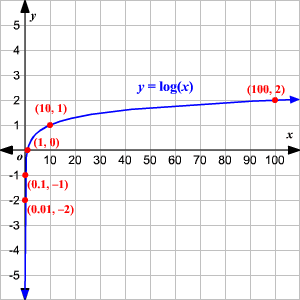# Graphing Exponential and Logarithmic Functions

## Graphing Exponential Functions

A simple exponential function to graph is $y={2}^{x}$ .

 $x$ $-3$ $-2$ $-1$ $0$ $1$ $2$ $3$ $y={2}^{x}$ $\frac{1}{8}$ $\frac{1}{4}$ $\frac{1}{2}$ $1$ $2$ $4$ $8$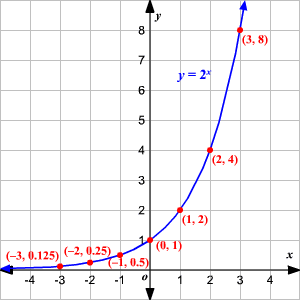Notice that the graph has the $x$ -axis as an asymptote on the left, and increases very fast on the right.

Changing the base changes the shape of the graph.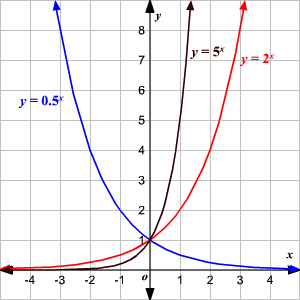Replacing $x$ with $-x$ reflects the graph across the $y$ -axis; replacing $y$ with $-y$ reflects it across the $x$ -axis.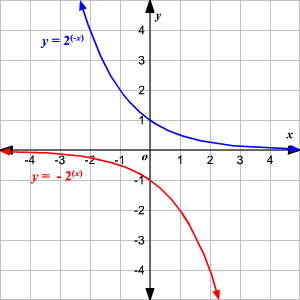Replacing $x$ with $x+h$ translates the graph $h$ units to the left.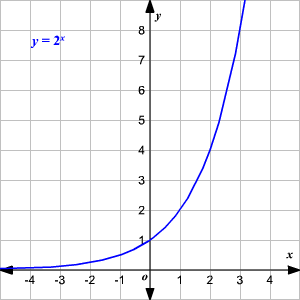Replacing $y$ with $y-k$ (which is the same as adding $k$ to the right side) translates the graph $k$ units up.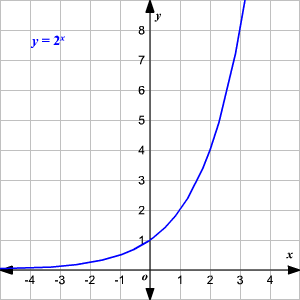## Graphing Logarithmic Functions

The function $y={\mathrm{log}}_{b}x$ is the inverse function of $y={b}^{x}$ . So, it is the reflection of that graph across the diagonal line $y=x$ .

When no base is written, assume that the log is base $10$ .

 $x$ $\frac{1}{1000}$ $\frac{1}{100}$ $\frac{1}{10}$ $1$ $10$ $100$ $1000$ $y=\mathrm{log}x$ $-3$ $-2$ $-1$ $0$ $1$ $2$ $3$